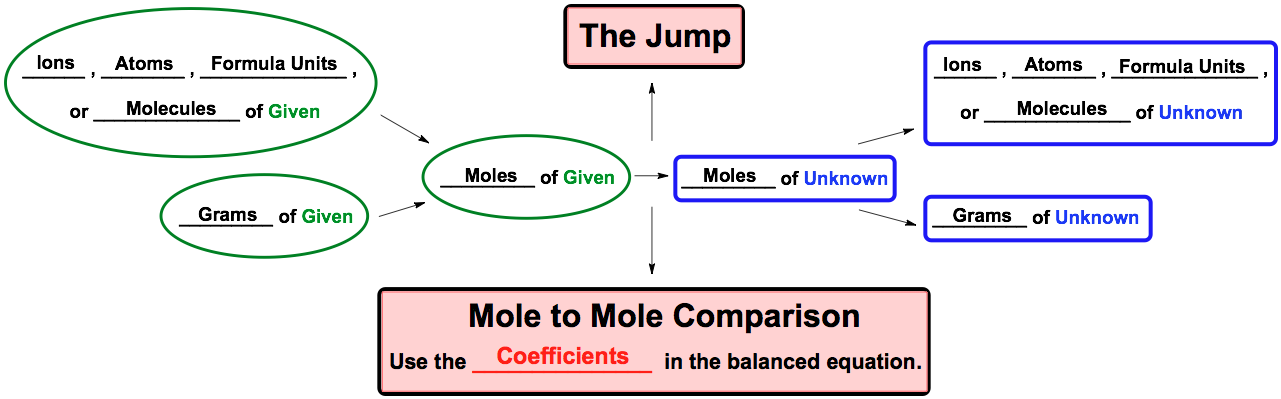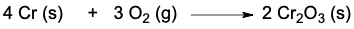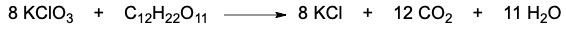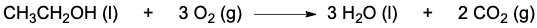Clutch Prep is now a part of Pearson
Ch.6 Chemical Reactions & QuantitiesWorksheetSee all chapters

# Stoichiometry

See all sections
Sections
Empirical Formula
Molecular Formula
Calculating Molar Mass
Mole Concept
Mass Percent
Stoichiometry
Limiting Reagent
Percent Yield

Stoichiometry deals with the numerical relationship between compounds in a balanced chemical equation.

###### Stoichiometry

Concept #1: Stoichiometry

Concept #2: Stoichiometric Chart

Utilize the stoichiometric chart to do all your stoichiometric calculations.Example #1: How many grams of H2O are produced when 12.3 g H2 reacts?

2 H2 (g) + 1 O2 (g) → 2 H2O (g)

Practice: The oxidation of chromium solid is represented by the following equation:How many moles of chromium (III) oxide are produced when 34.69 g Cr reacts with excess oxygen gas?

Practice: The reaction of potassium chlorate and sucrose is given below:If 2.33 x 10-7 formula units of potassium chlorate are reacted, how many grams of carbon dioxide will be produced?

Practice: If the density of ethanol, CH3CH2OH, is 0.789 g/mL, how many milliliters of ethanol are needed to produce 4.8 g of H2O in the following reaction?